The univar_numeric() calculates statistic of numerical variables that is frequency table

univar_numeric(.data, ...)

# S3 method for data.frame
univar_numeric(.data, ...)

## Arguments

.data a data.frame or a tbl_df. one or more unquoted expressions separated by commas. You can treat variable names like they are positions. Positive values select variables; negative values to drop variables. These arguments are automatically quoted and evaluated in a context where column names represent column positions. They support unquoting and splicing.

## Value

An object of the class as individual variables based list. A component named "statistics" is a tibble object with the following statistics.:

• variable : factor. The level of the variable. 'variable' is the name of the variable.

• n : number of observations excluding missing values

• na : number of missing values

• mean : arithmetic average

• sd : standard deviation

• se_mean : standrd error mean. sd/sqrt(n)

• IQR : interquartile range (Q3-Q1)

• skewness : skewness

• kurtosis : kurtosis

• median : median. 50% percentile

## Details

univar_numeric() calculates the popular statistics of numerical variables. If a specific variable name is not specified, statistics for all categorical numerical included in the data are calculated. The statistics obtained by univar_numeric() are part of those obtained by describe(). Therefore, it is recommended to use describe() to simply calculate statistics. However, if you want to visualize the distribution of individual variables, you should use univar_numeric().

## Attributes of return object

Attributes of compare_category class is as follows.

• raw : a data.frame or a tbl_df. Data containing variables to be compared. Save it for visualization with plot.univar_numeric().

• variables : character. List of variables selected for calculate statistics.

summary.univar_numeric, print.univar_numeric, plot.univar_numeric.

## Examples

# \donttest{
# Calculates the all categorical variables
all_var <- univar_numeric(heartfailure)

# Print univar_numeric class object
all_var#> $statistics #> # A tibble: 7 x 10 #> variable n na mean sd se_mean IQR skewness kurtosis median #> <chr> <int> <int> <dbl> <dbl> <dbl> <dbl> <dbl> <dbl> <dbl> #> 1 age 299 0 6.08e1 1.19e1 6.88e-1 19 0.424 -0.184 6 e1 #> 2 cpk_enzyme 299 0 5.82e2 9.70e2 5.61e+1 466. 4.46 25.1 2.5 e2 #> 3 ejection_… 299 0 3.81e1 1.18e1 6.84e-1 15 0.555 0.0414 3.8 e1 #> 4 platelets 299 0 2.63e5 9.78e4 5.66e+3 91000 1.46 6.21 2.62e5 #> 5 creatinine 299 0 1.39e0 1.03e0 5.98e-2 0.5 4.46 25.8 1.1 e0 #> 6 sodium 299 0 1.37e2 4.41e0 2.55e-1 6 -1.05 4.12 1.37e2 #> 7 time 299 0 1.30e2 7.76e1 4.49e+0 130 0.128 -1.21 1.15e2 #> # Calculates the platelets, sodium variable univar_numeric(heartfailure, platelets, sodium)#>$statistics
#> # A tibble: 2 x 10
#>   variable      n    na    mean       sd  se_mean   IQR skewness kurtosis median
#>   <chr>     <int> <int>   <dbl>    <dbl>    <dbl> <dbl>    <dbl>    <dbl>  <dbl>
#> 1 platelets   299     0 263358. 97804.   5656.    91000     1.46     6.21 262000
#> 2 sodium      299     0    137.     4.41    0.255     6    -1.05     4.12    137
#>
# Summary the all case : Return a invisible copy of an object.
stat <- summary(all_var)

# Summary by returned object
stat#> # A tibble: 7 x 8
#>   variable              mean    sd se_mean   IQR skewness kurtosis median
#>   <chr>                <dbl> <dbl>   <dbl> <dbl>    <dbl>    <dbl>  <dbl>
#> 1 age                0.0437  0.626  0.0362     1    0.424  -0.184       0
#> 2 cpk_enzyme         0.713   2.08   0.121      1    4.46   25.1         0
#> 3 ejection_fraction  0.00557 0.789  0.0456     1    0.555   0.0414      0
#> 4 platelets          0.0149  1.07   0.0622     1    1.46    6.21        0
#> 5 creatinine         0.588   2.07   0.120      1    4.46   25.8         0
#> 6 sodium            -0.0624  0.735  0.0425     1   -1.05    4.12        0
#> 7 time               0.117   0.597  0.0345     1    0.128  -1.21        0
# Statistics of numerical variables normalized by Min-Max method
summary(all_var, stand = "minmax")#> # A tibble: 7 x 8
#>   variable            mean    sd se_mean    IQR skewness kurtosis median
#>   <chr>              <dbl> <dbl>   <dbl>  <dbl>    <dbl>    <dbl>  <dbl>
#> 1 age               0.379  0.216 0.0125  0.345     0.424  -0.184  0.364
#> 2 cpk_enzyme        0.0713 0.124 0.00716 0.0594    4.46   25.1    0.0290
#> 3 ejection_fraction 0.365  0.179 0.0104  0.227     0.555   0.0414 0.364
#> 4 platelets         0.289  0.119 0.00686 0.110     1.46    6.21   0.287
#> 5 creatinine        0.100  0.116 0.00672 0.0562    4.46   25.8    0.0674
#> 6 sodium            0.675  0.126 0.00729 0.171    -1.05    4.12   0.686
#> 7 time              0.449  0.276 0.0160  0.463     0.128  -1.21   0.395
# Statistics of numerical variables standardized by Z-score method
summary(all_var, stand = "zscore")#> # A tibble: 7 x 8
#>   variable               mean    sd se_mean   IQR skewness kurtosis   median
#>   <chr>                 <dbl> <dbl>   <dbl> <dbl>    <dbl>    <dbl>    <dbl>
#> 1 age                2.01e-16     1  0.0578 1.60     0.424  -0.184  -0.0697
#> 2 cpk_enzyme        -1.33e-17     1  0.0578 0.480    4.46   25.1    -0.342
#> 3 ejection_fraction -2.48e-17     1  0.0578 1.27     0.555   0.0414 -0.00706
#> 4 platelets          8.98e-17     1  0.0578 0.930    1.46    6.21   -0.0139
#> 5 creatinine        -7.88e-17     1  0.0578 0.483    4.46   25.8    -0.284
#> 6 sodium            -8.82e-16     1  0.0578 1.36    -1.05    4.12    0.0849
#> 7 time              -1.80e-16     1  0.0578 1.67     0.128  -1.21   -0.197
# one plot with all variables
plot(all_var)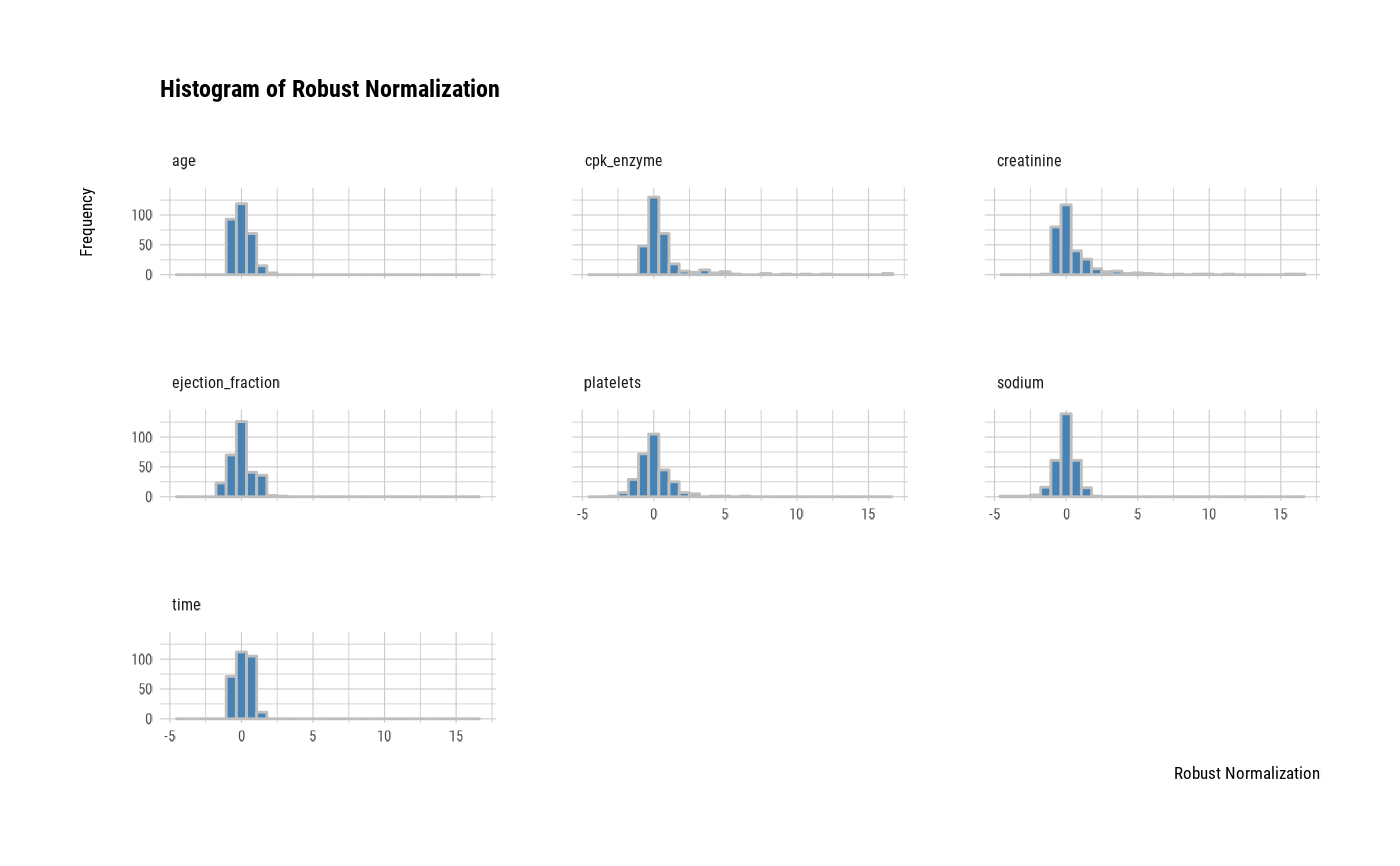# one plot with all normalized variables by Min-Max method
plot(all_var, stand = "minmax")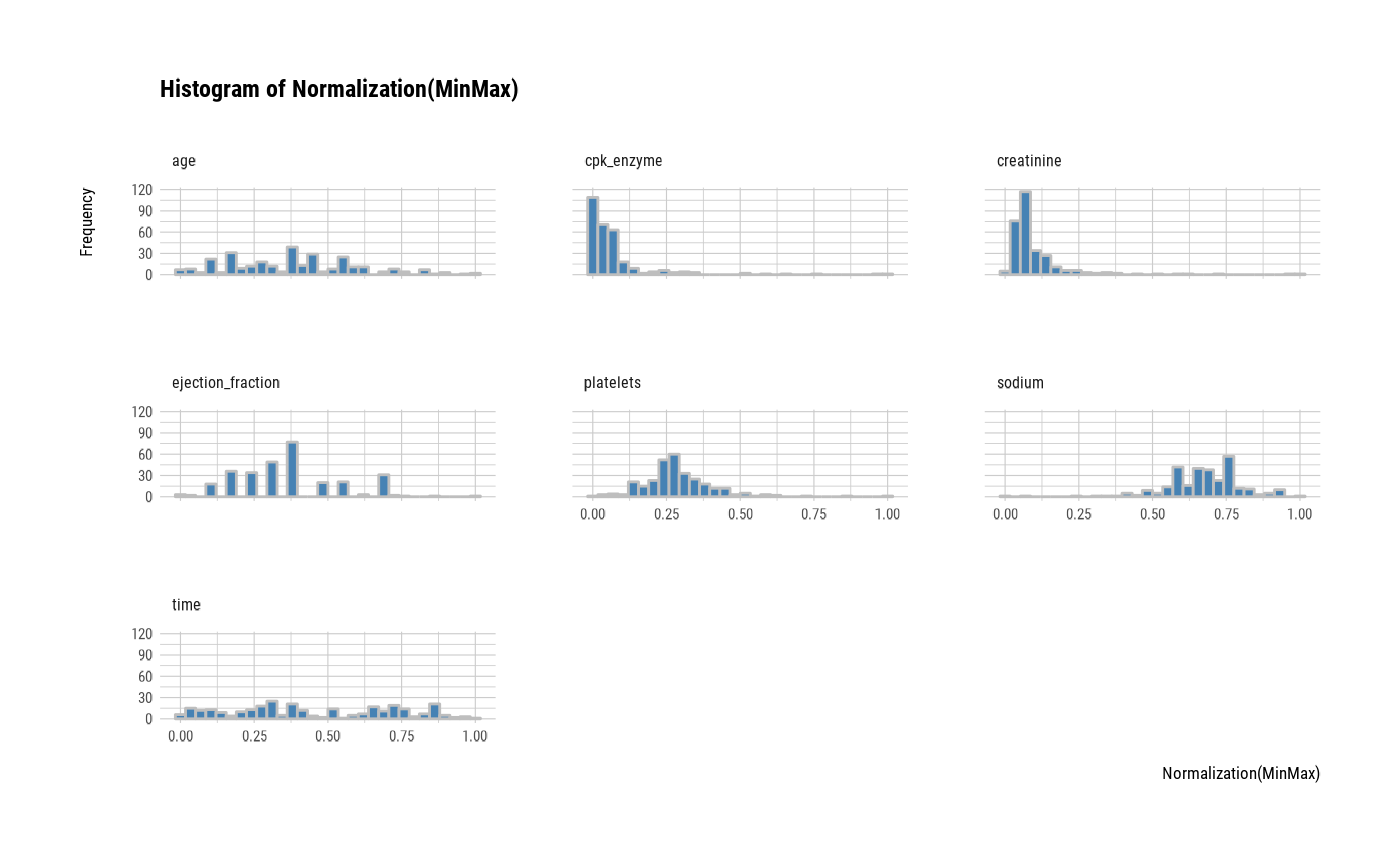# one plot with all variables
plot(all_var, stand = "none")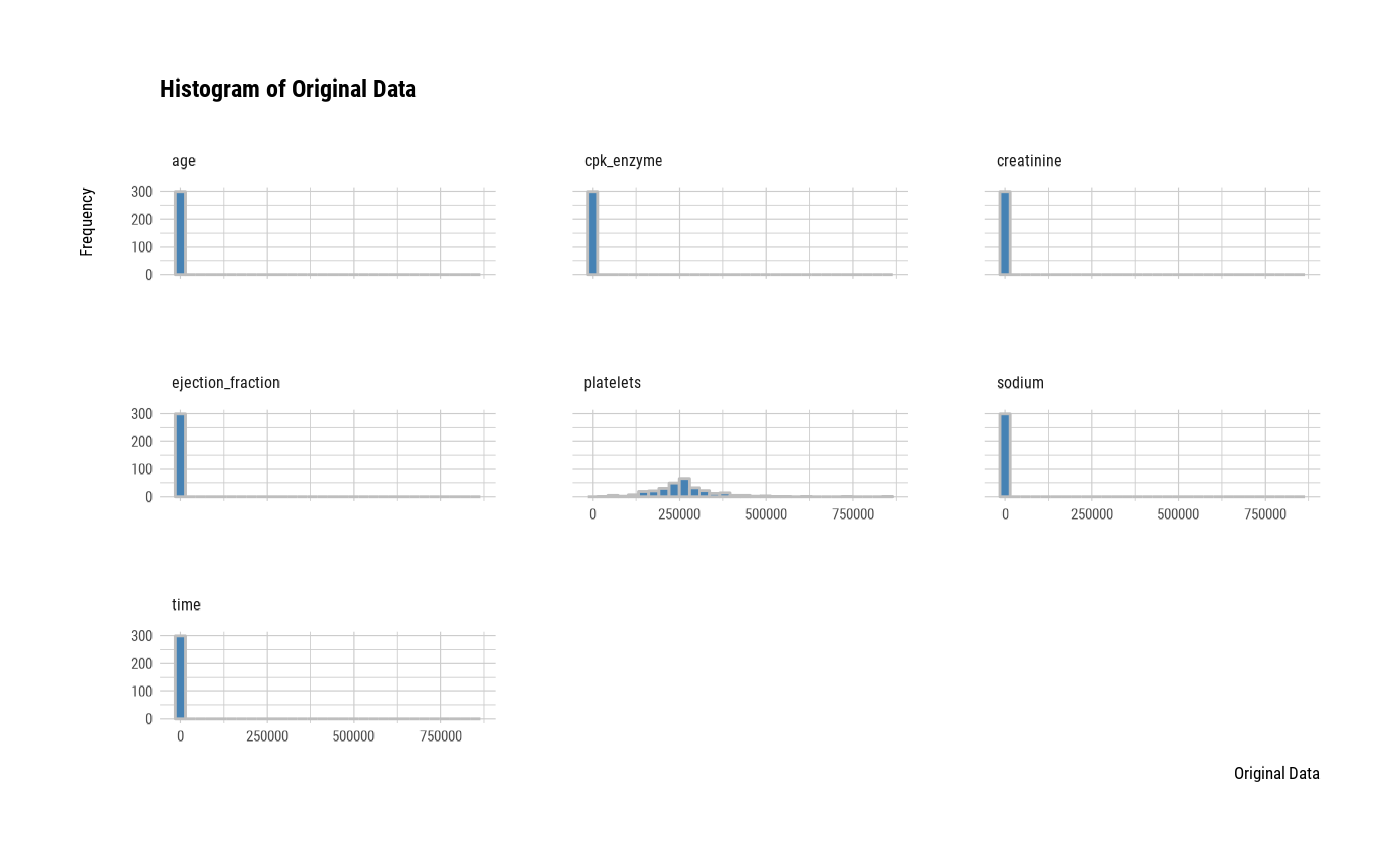# one plot with all robust standardized variables
plot(all_var, viz = "boxplot")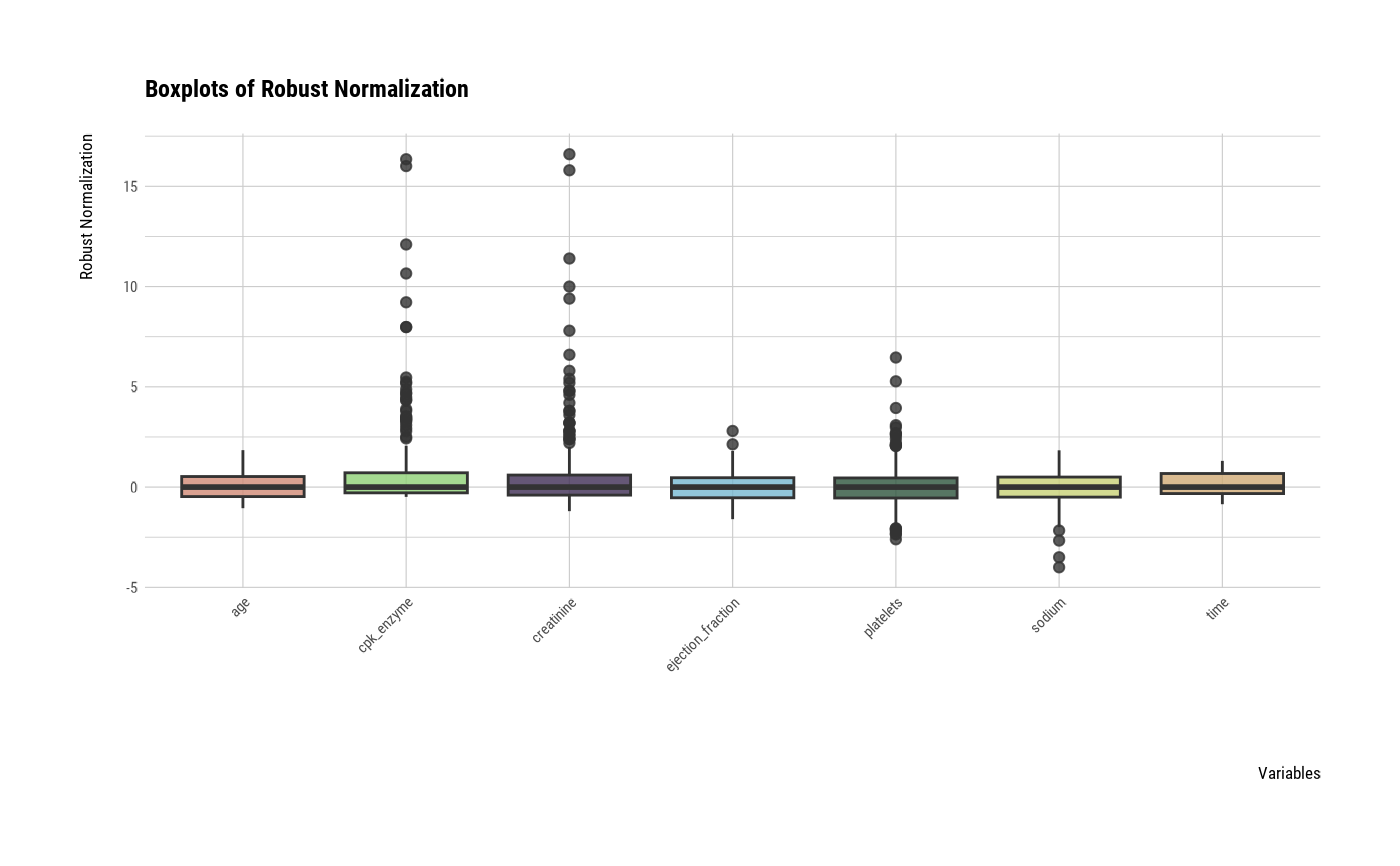# one plot with all standardized variables by Z-score method
plot(all_var, viz = "boxplot", stand = "zscore")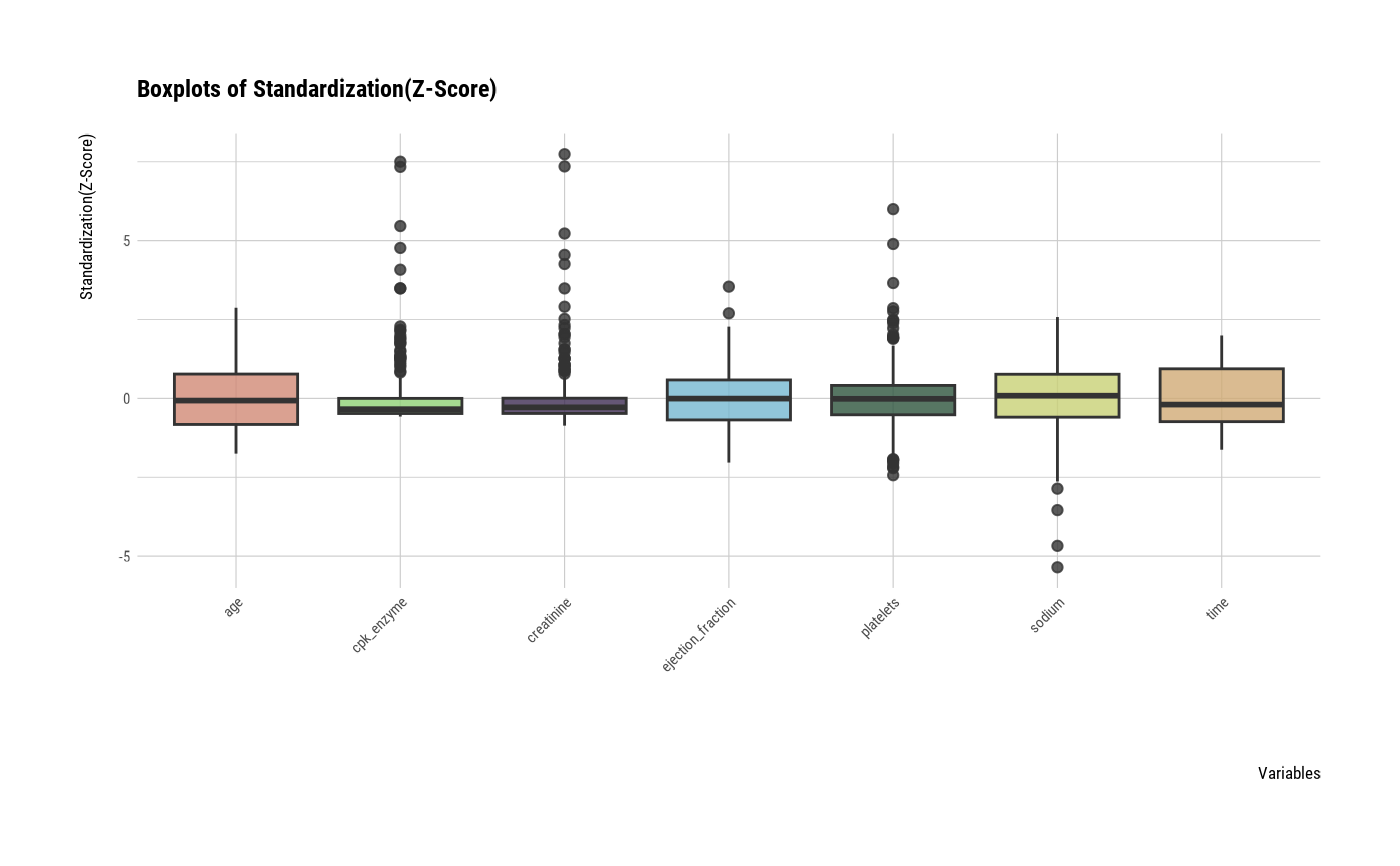# individual boxplot by variables
plot(all_var, indiv = TRUE, "boxplot")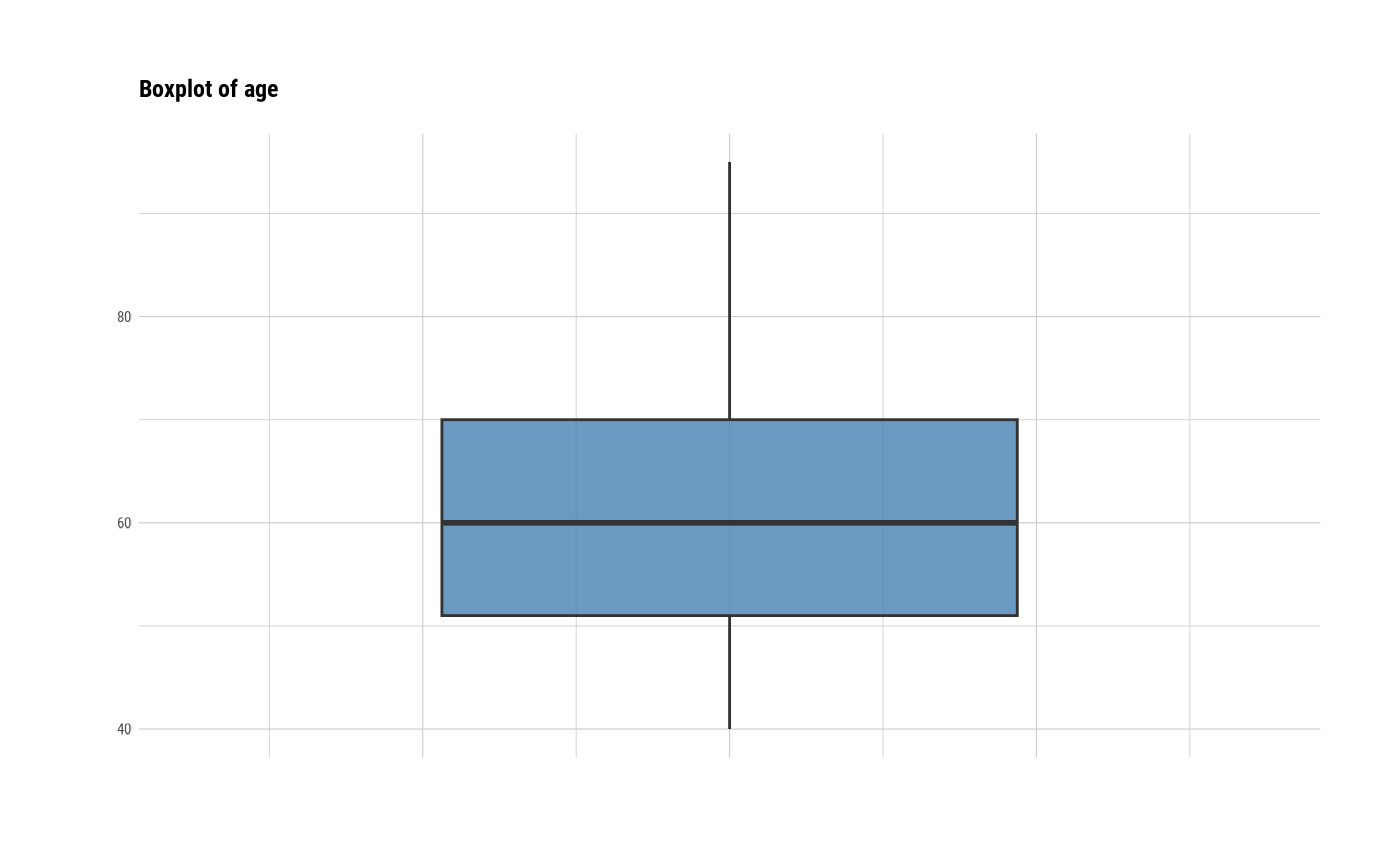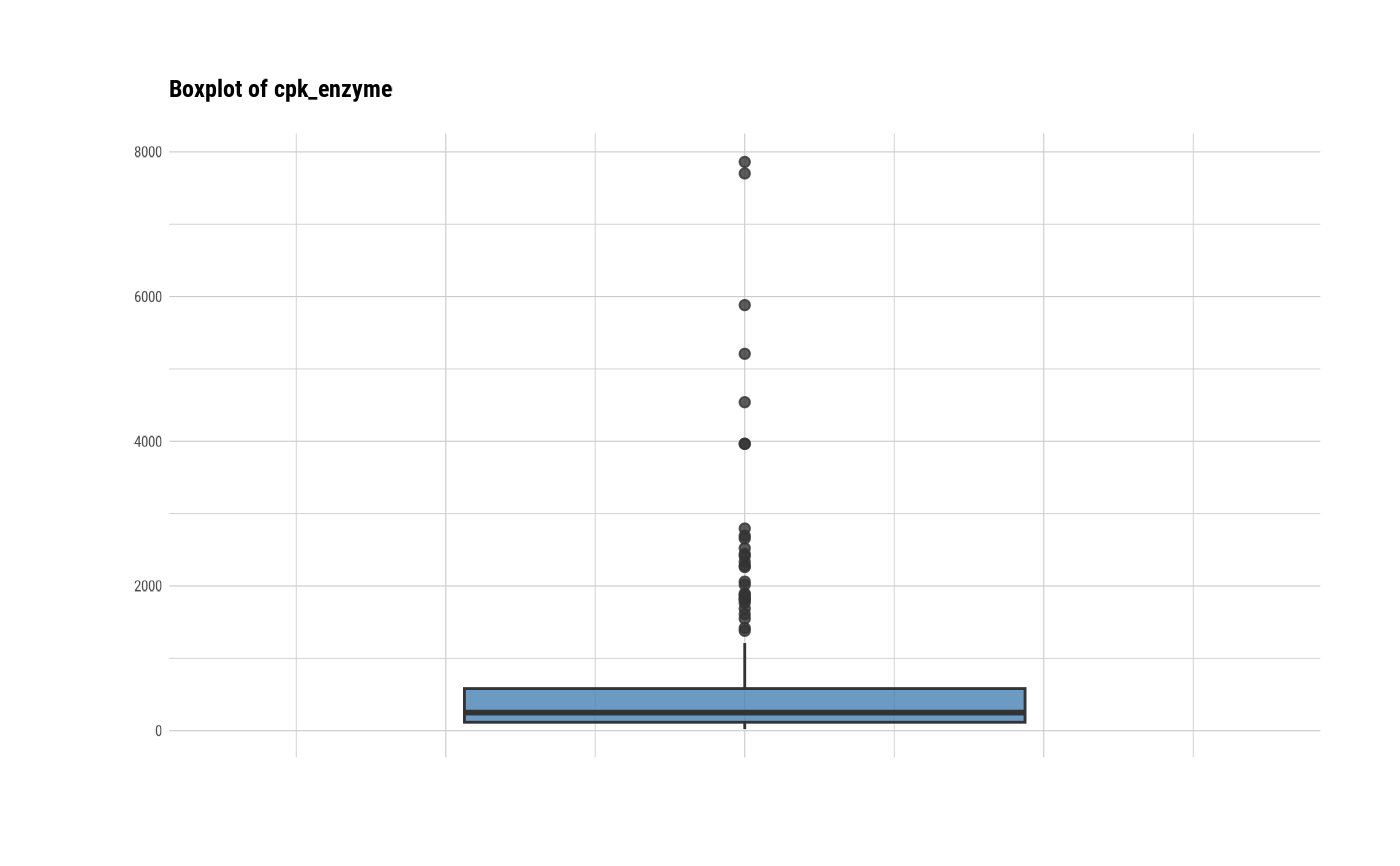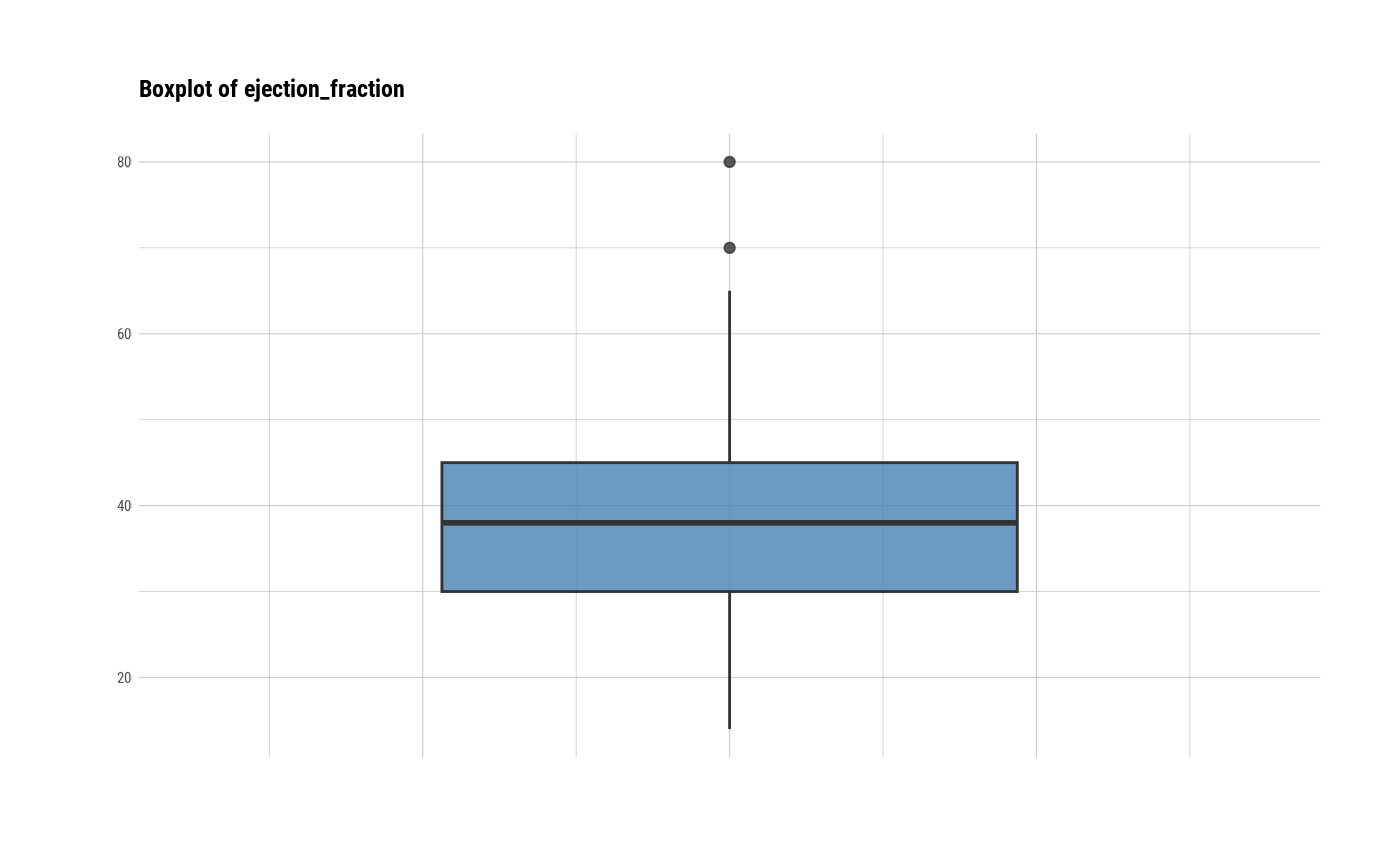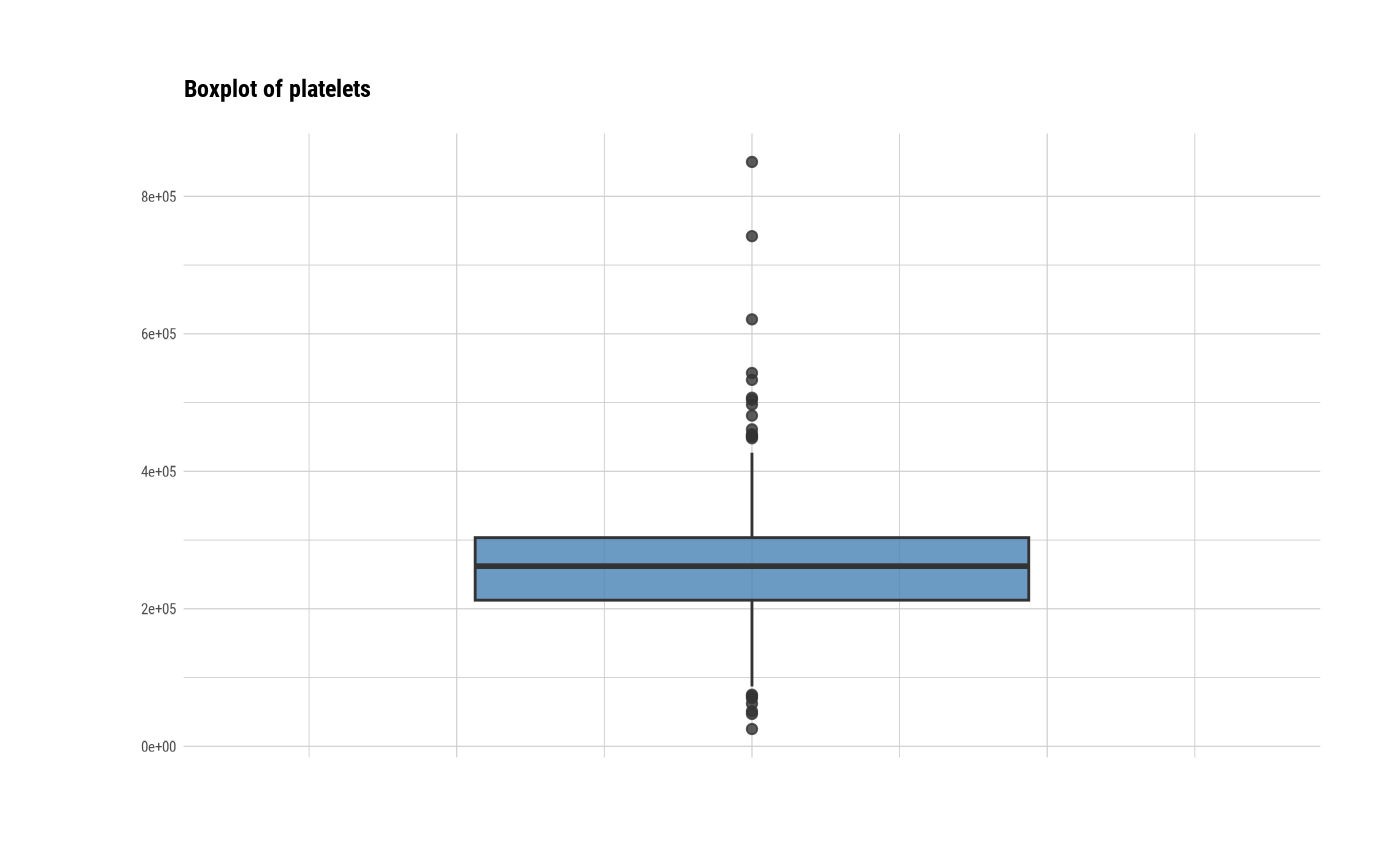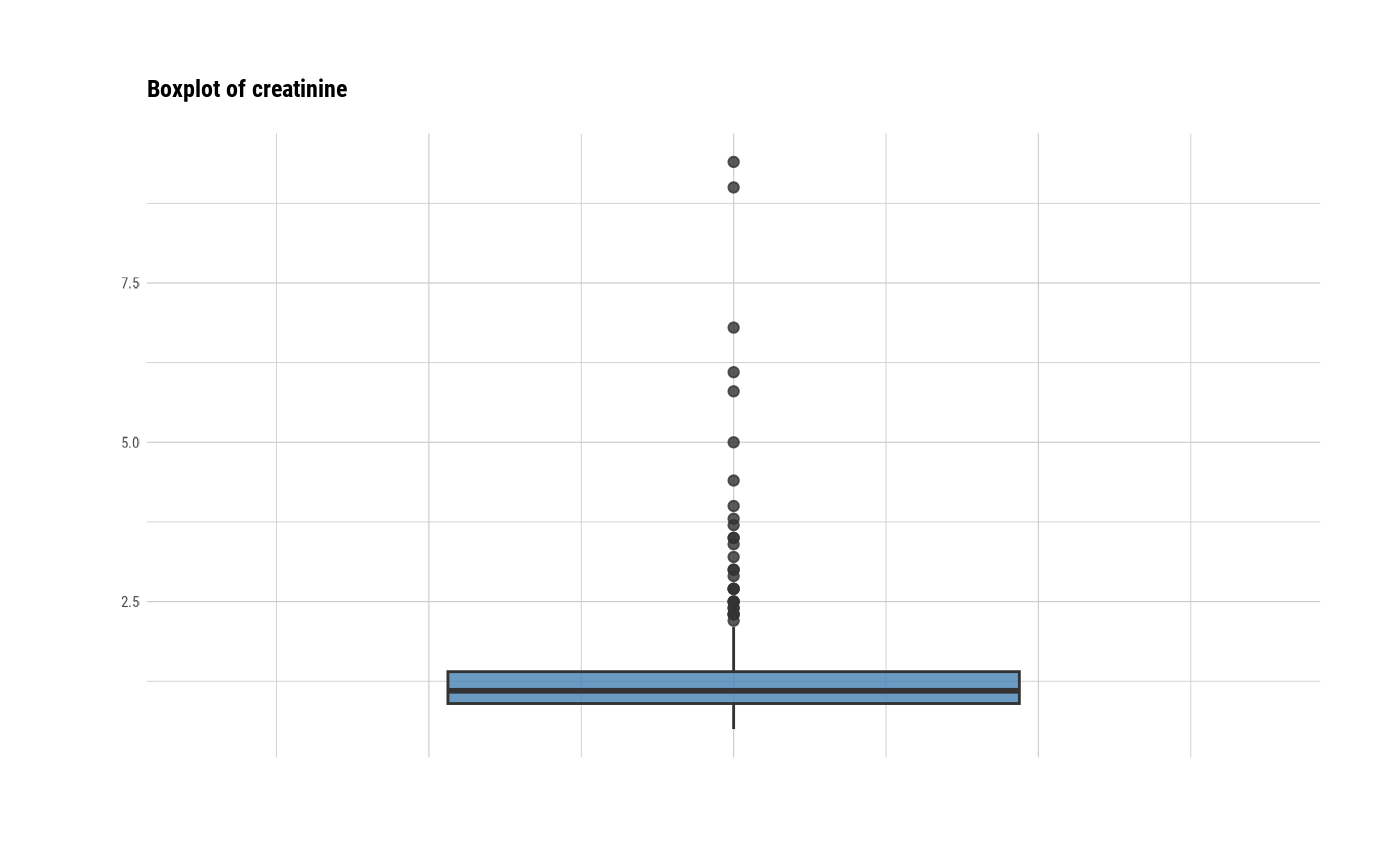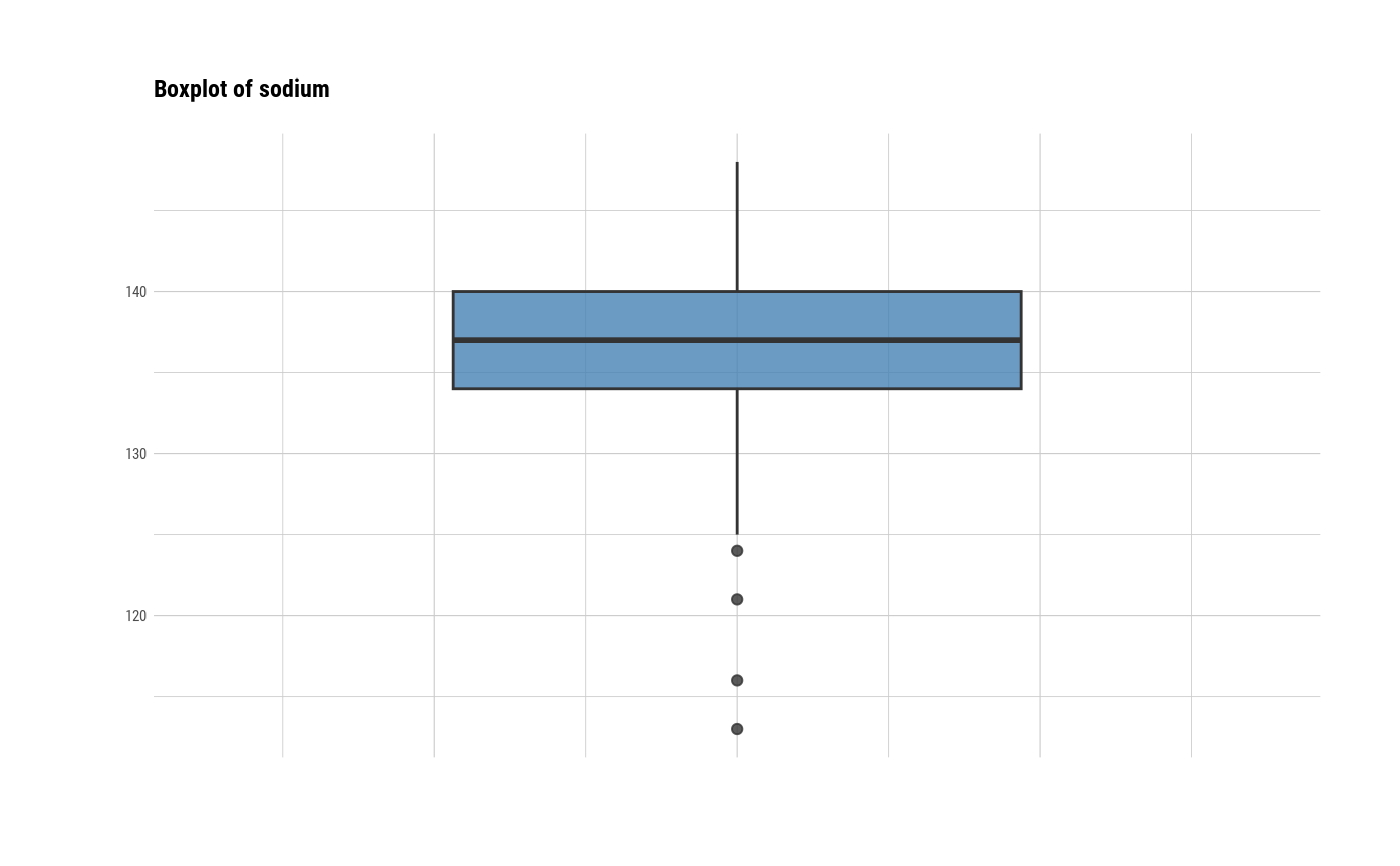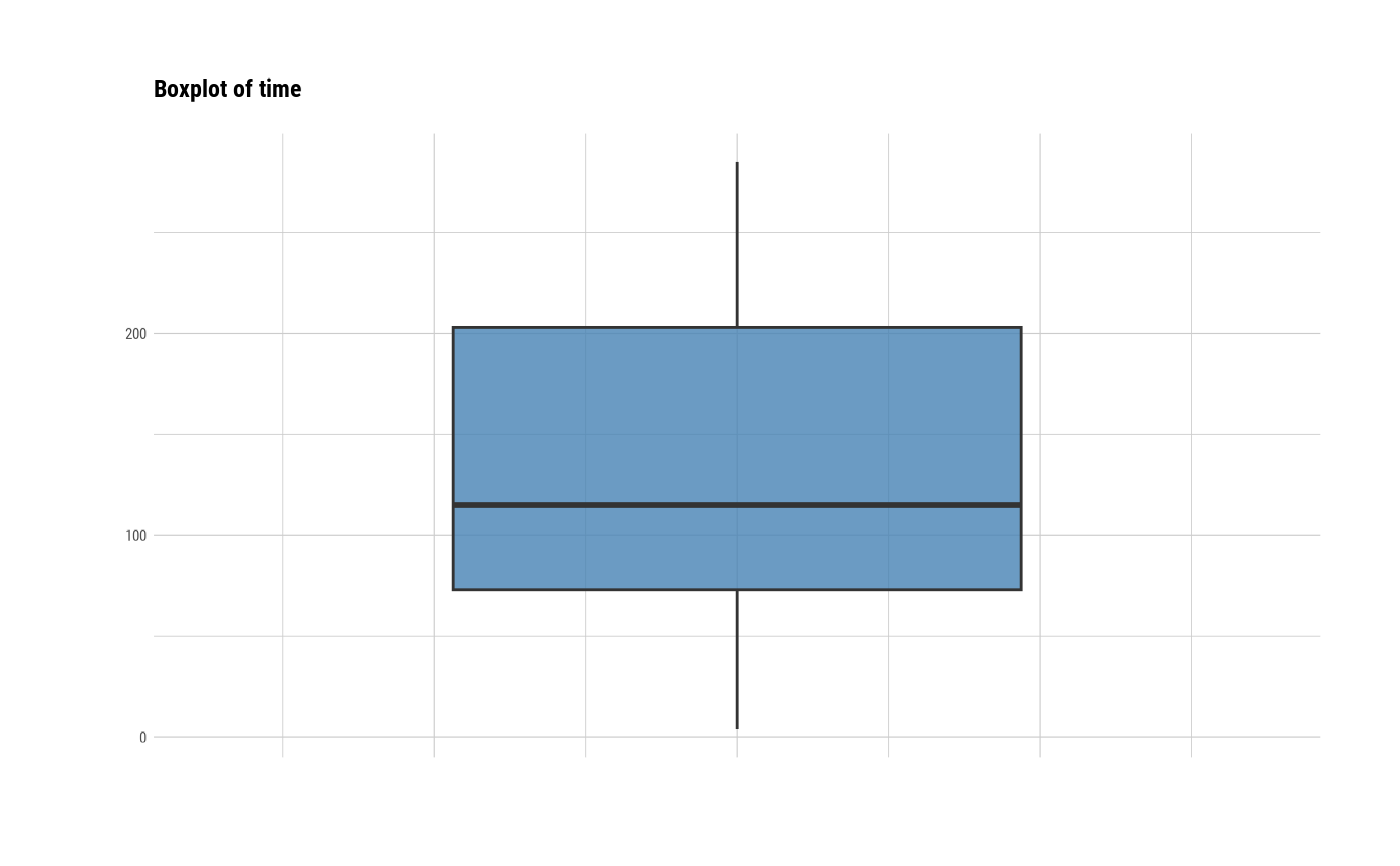# individual histogram by variables
plot(all_var, indiv = TRUE, "hist")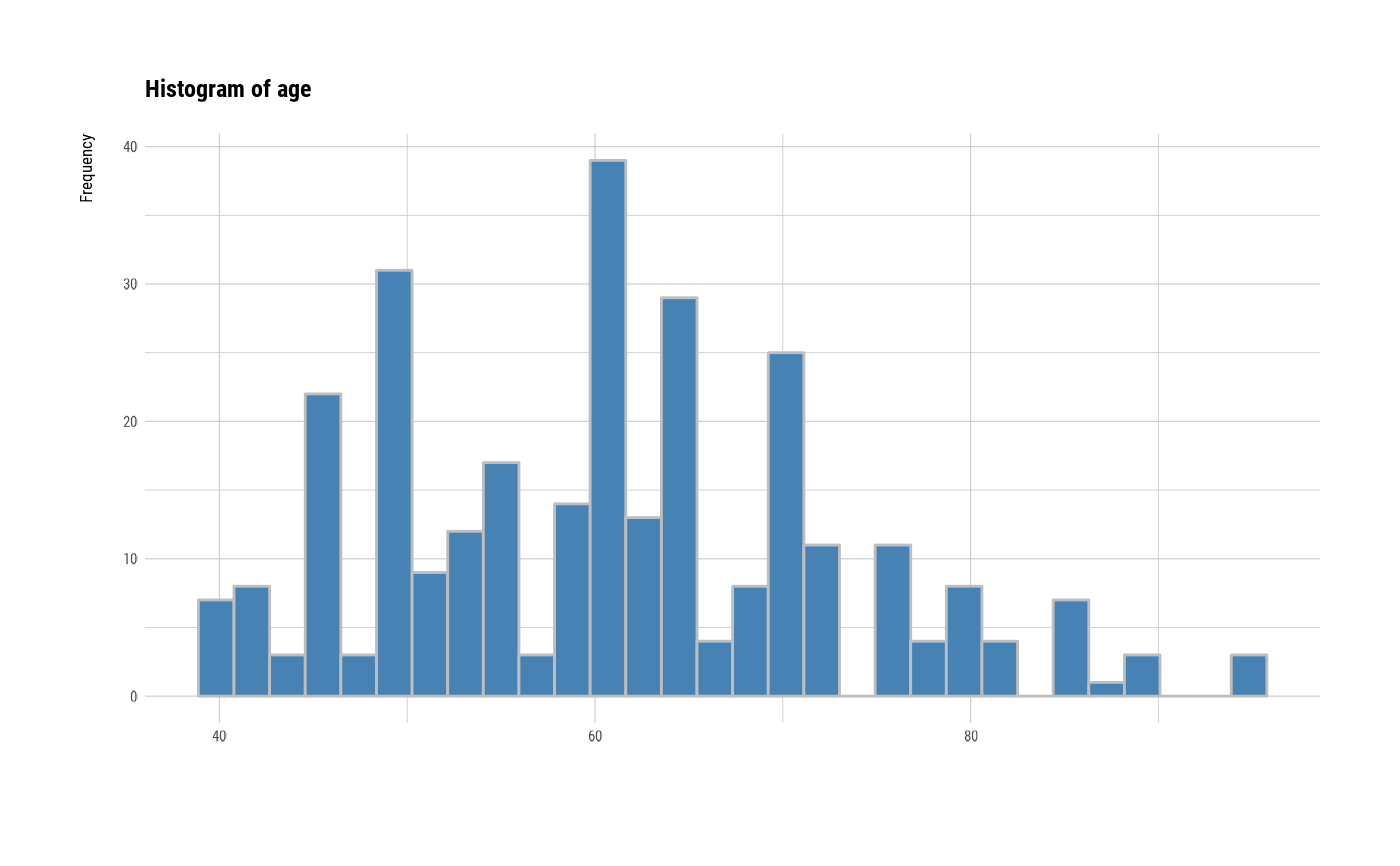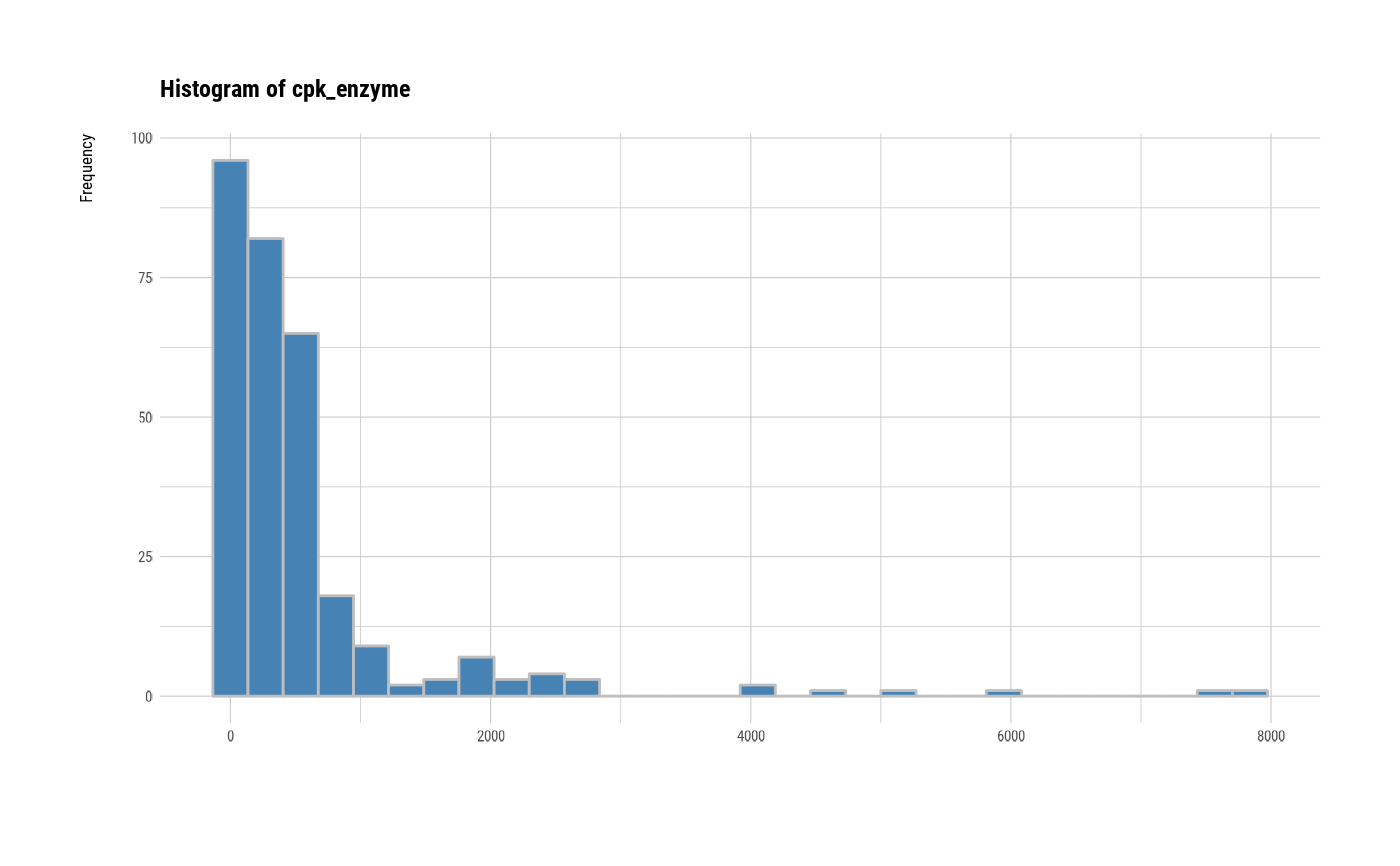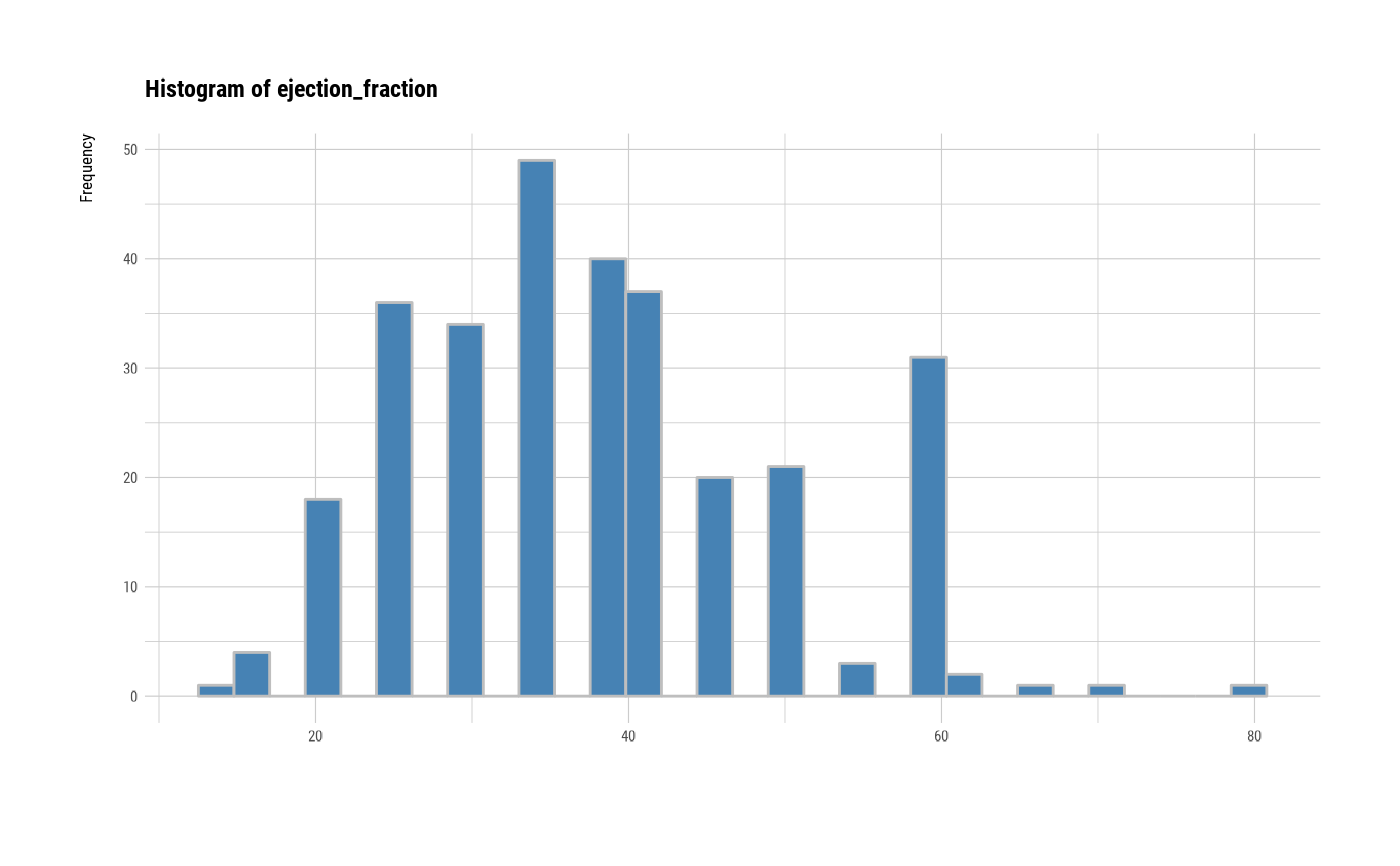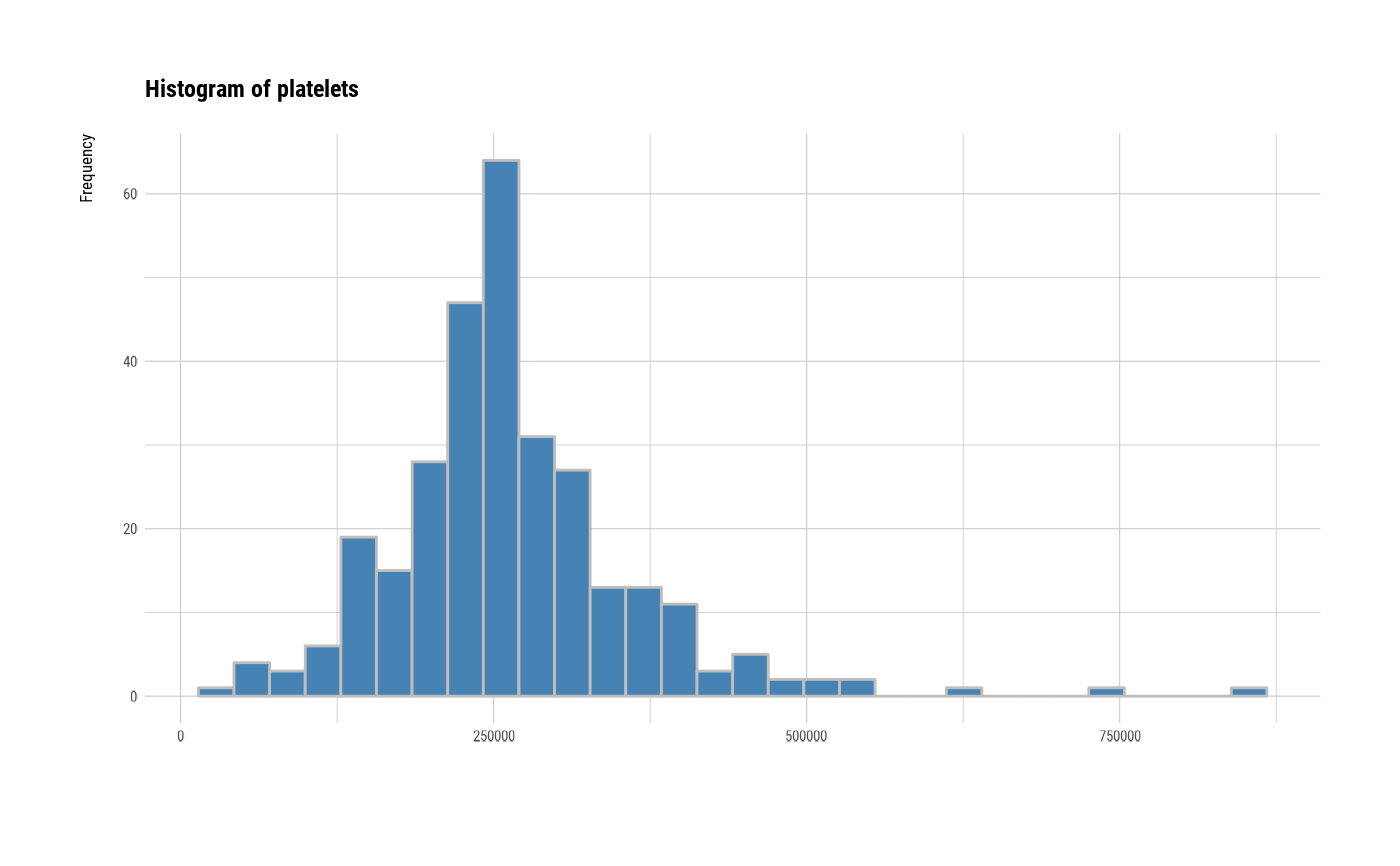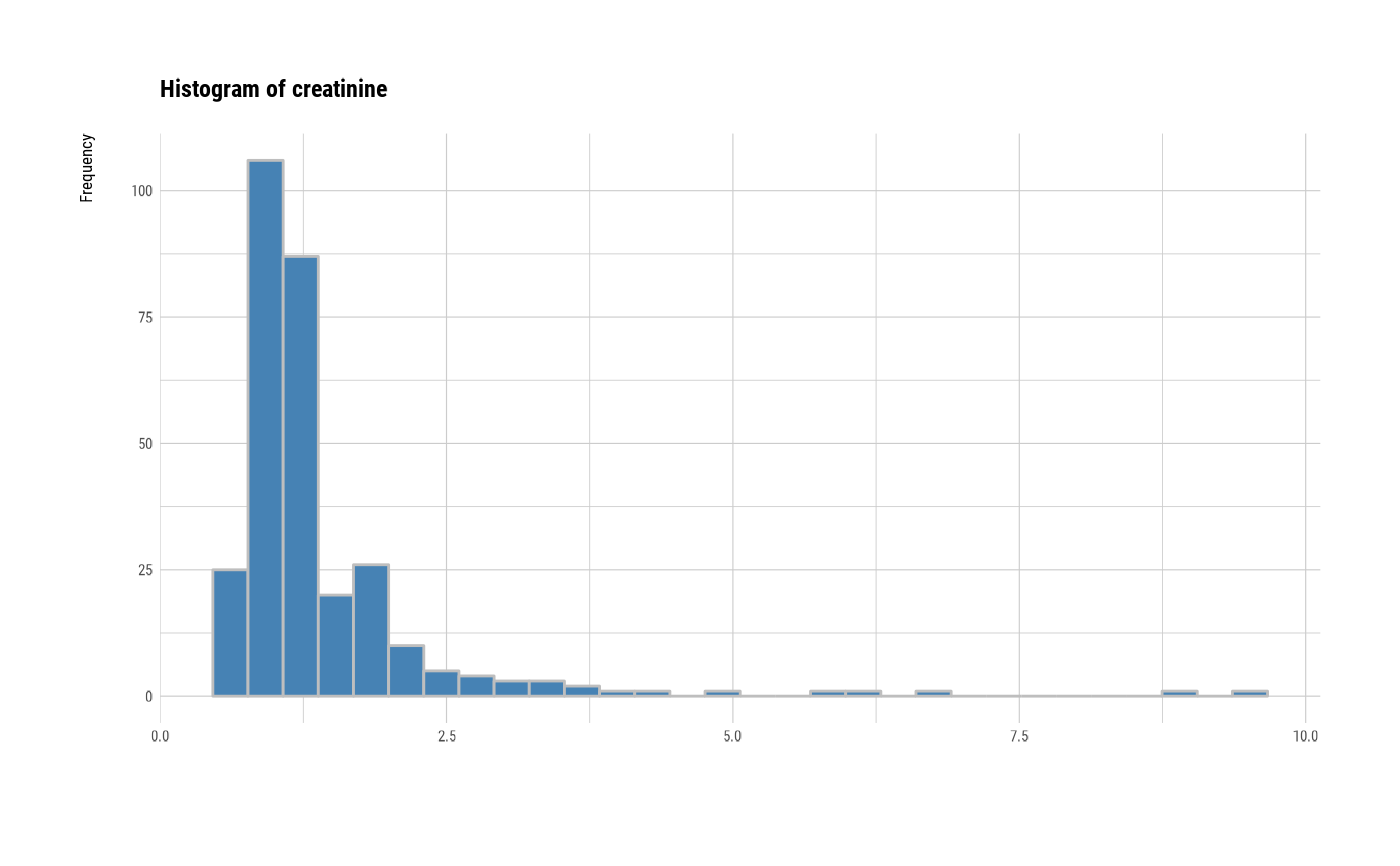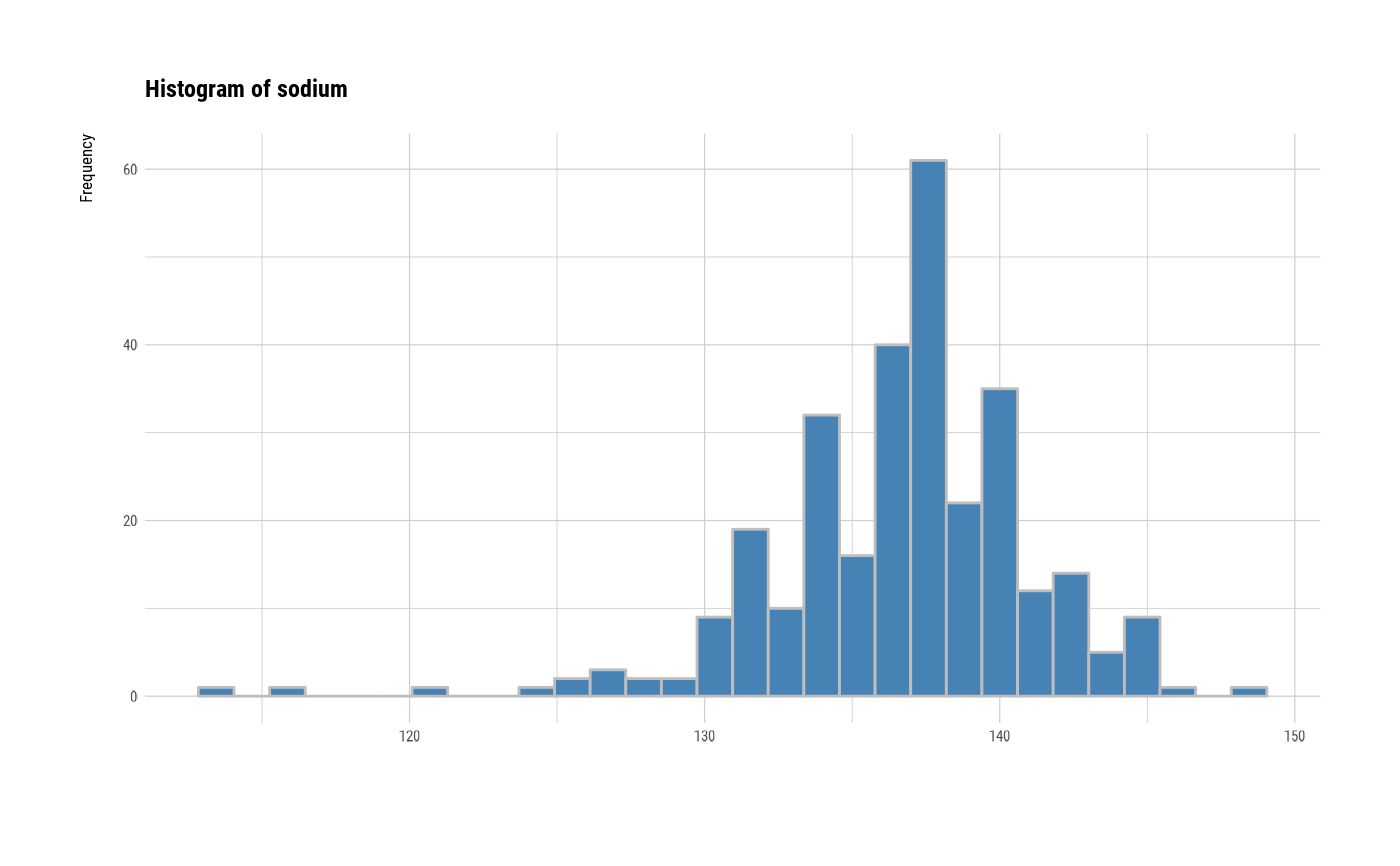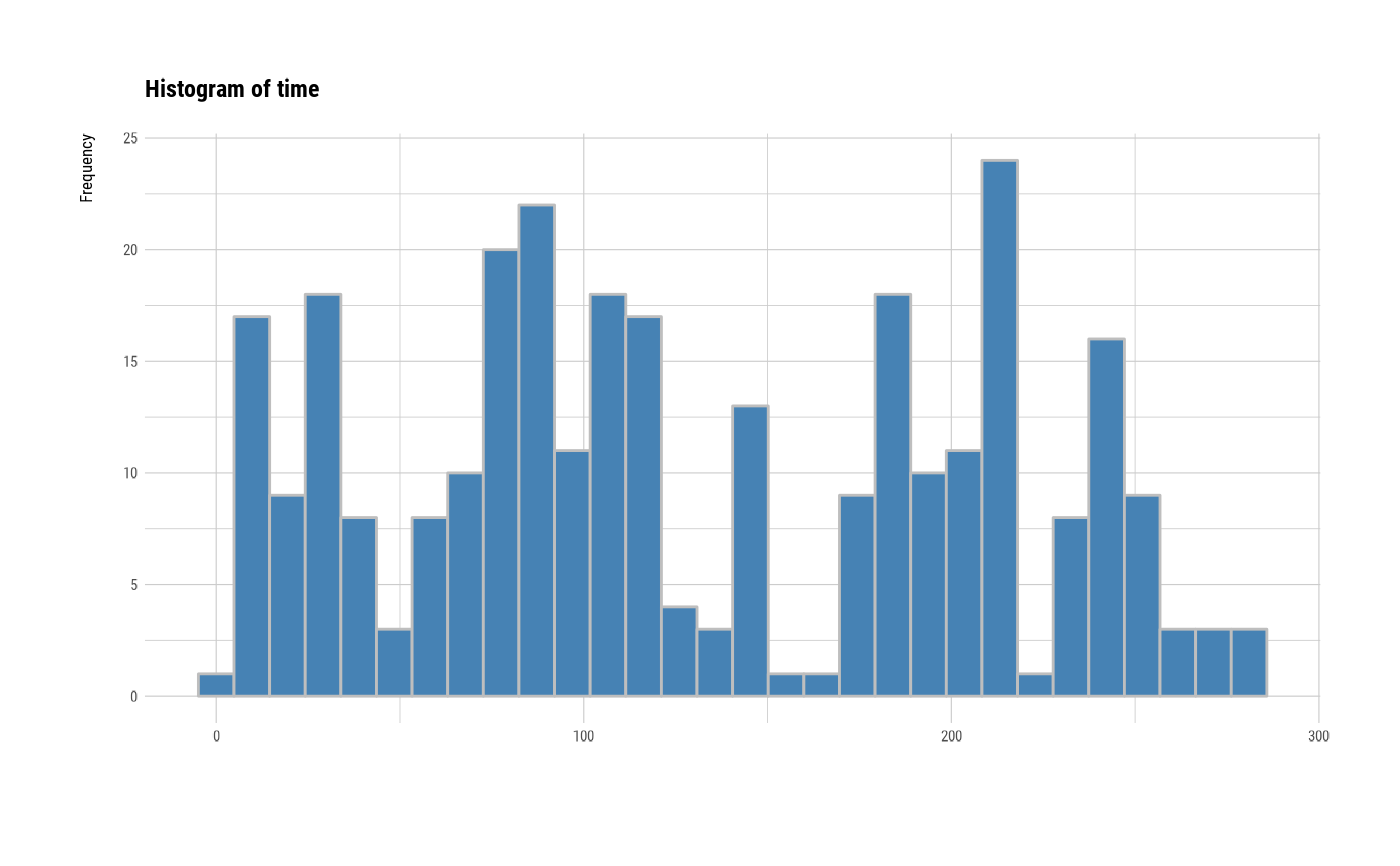# individual histogram by robust standardized variable
plot(all_var, indiv = TRUE, "hist", stand = "robust")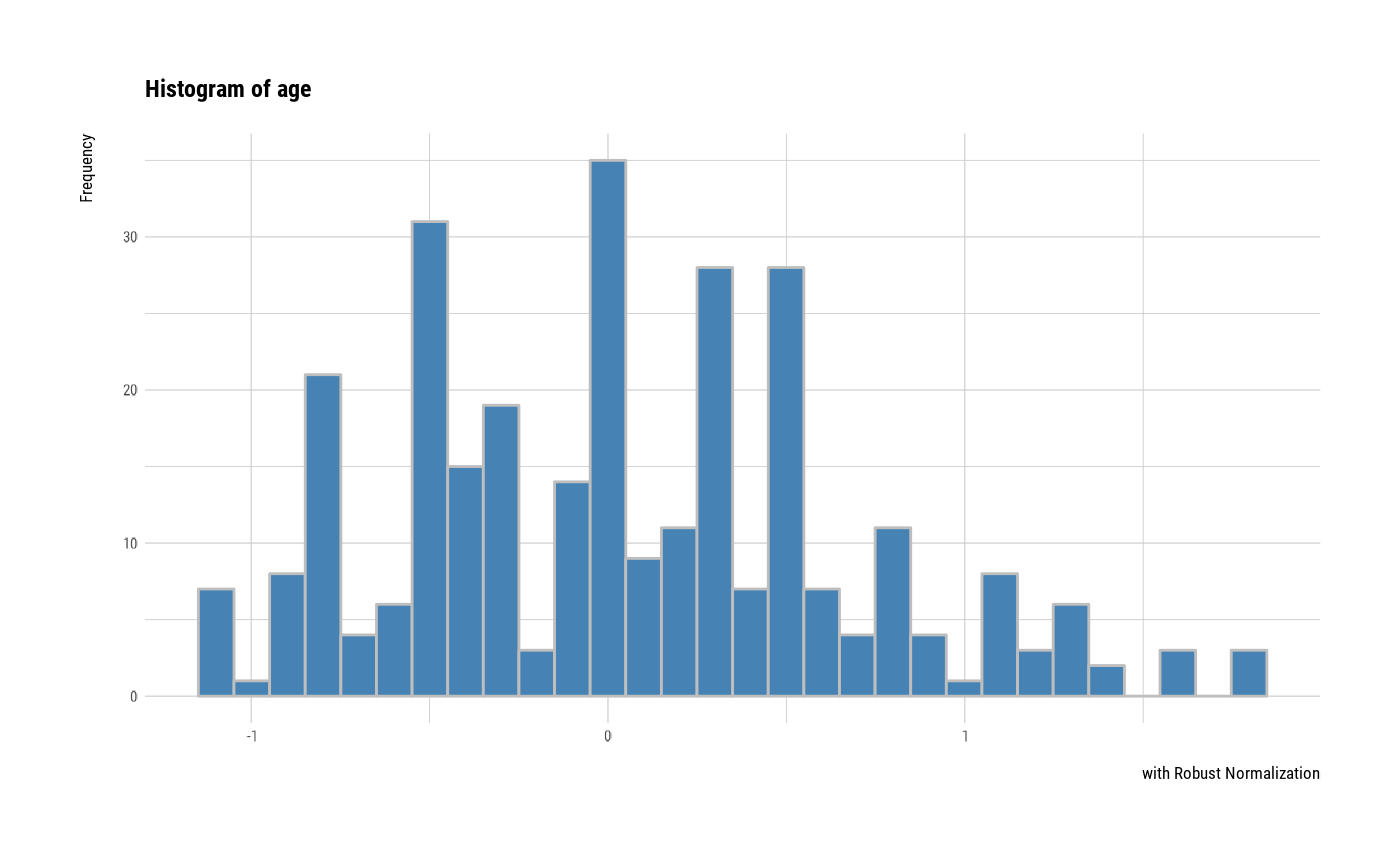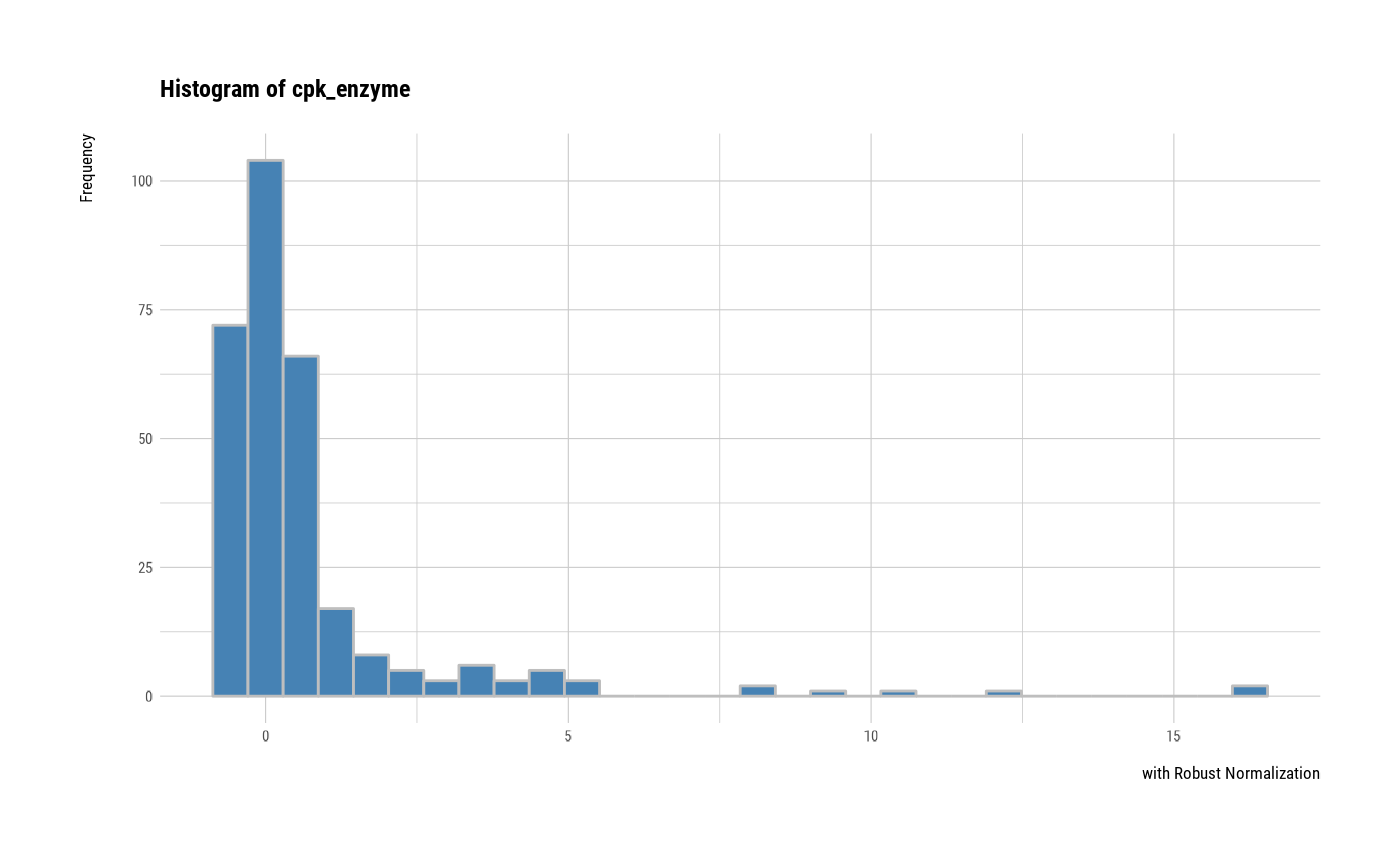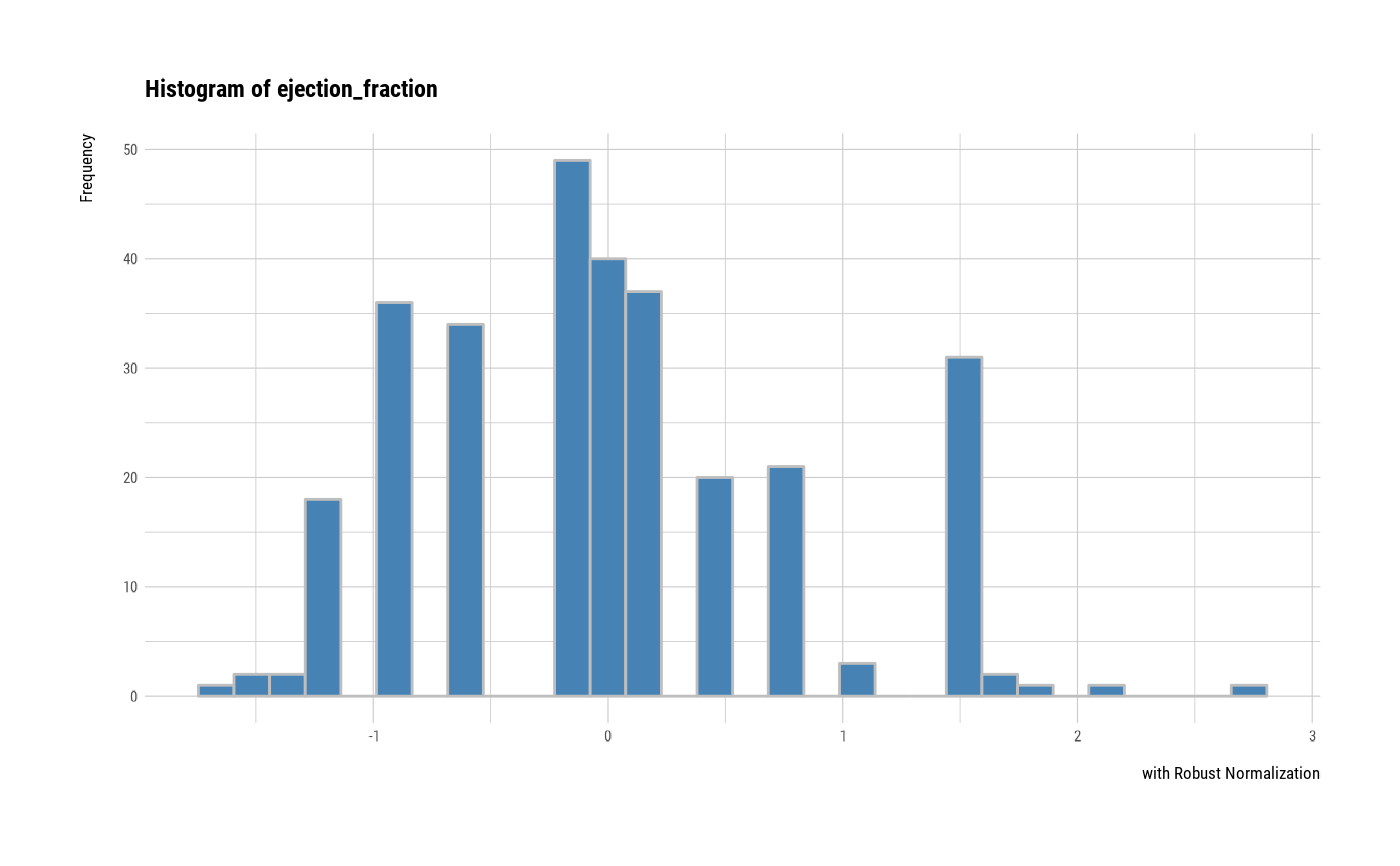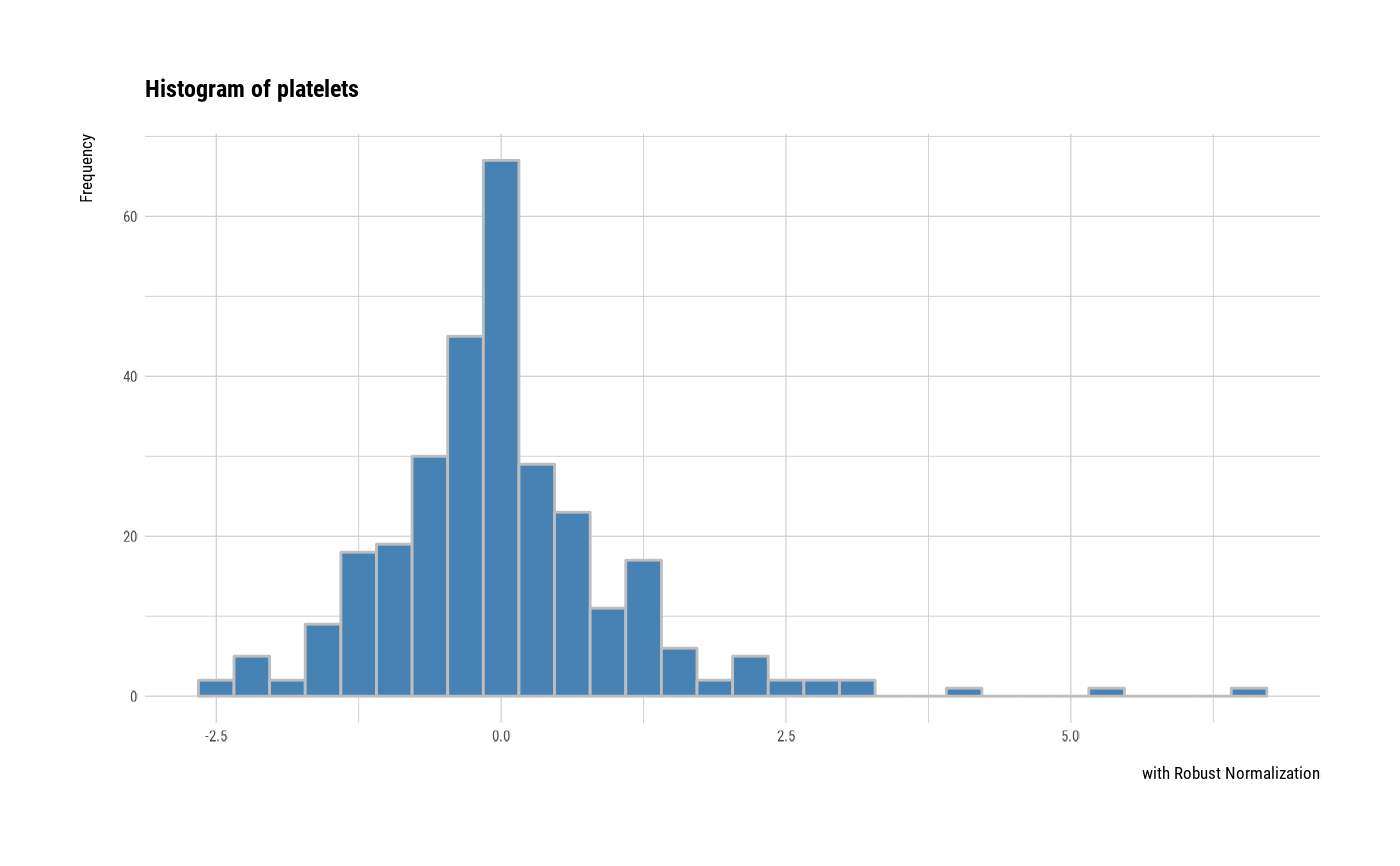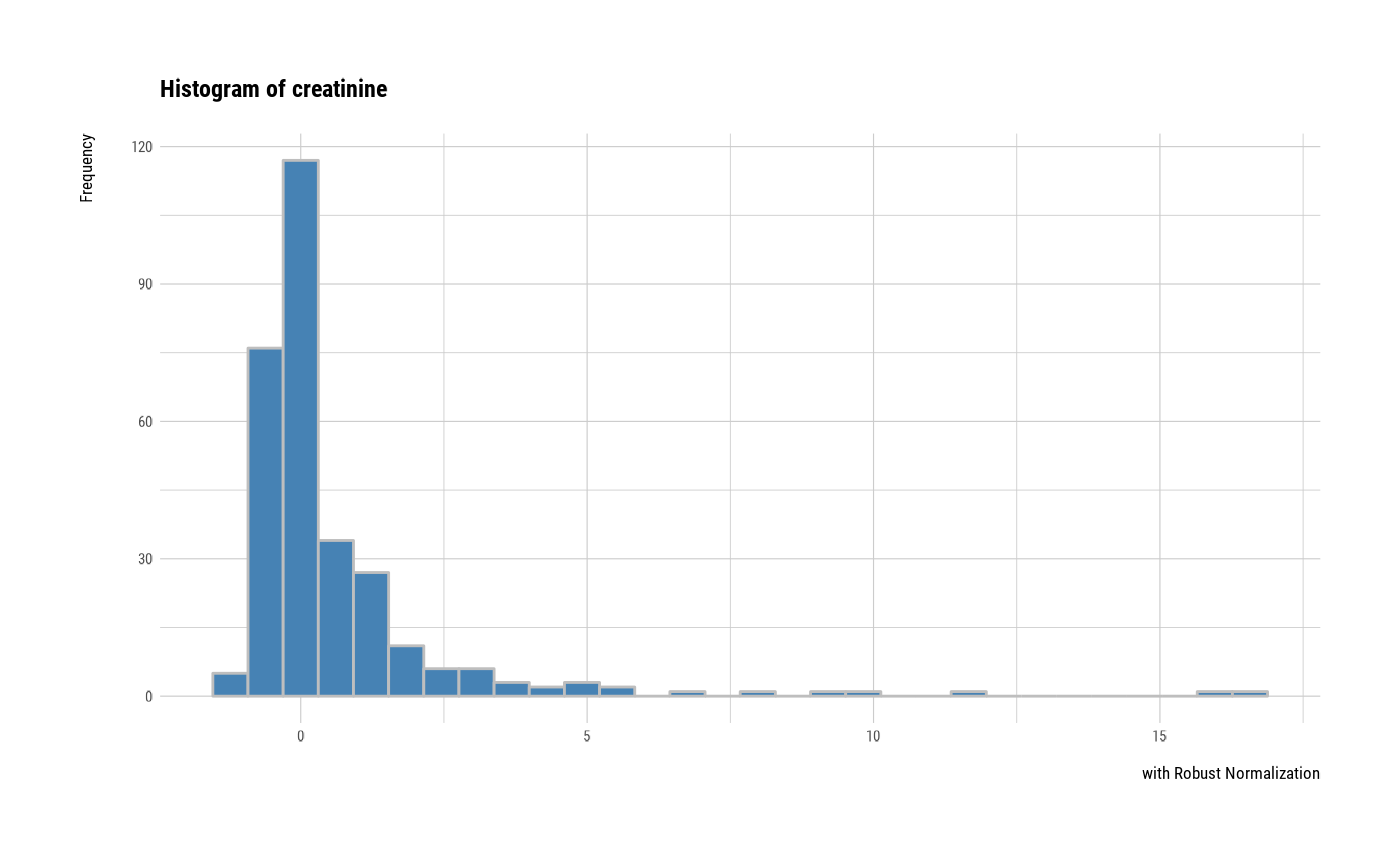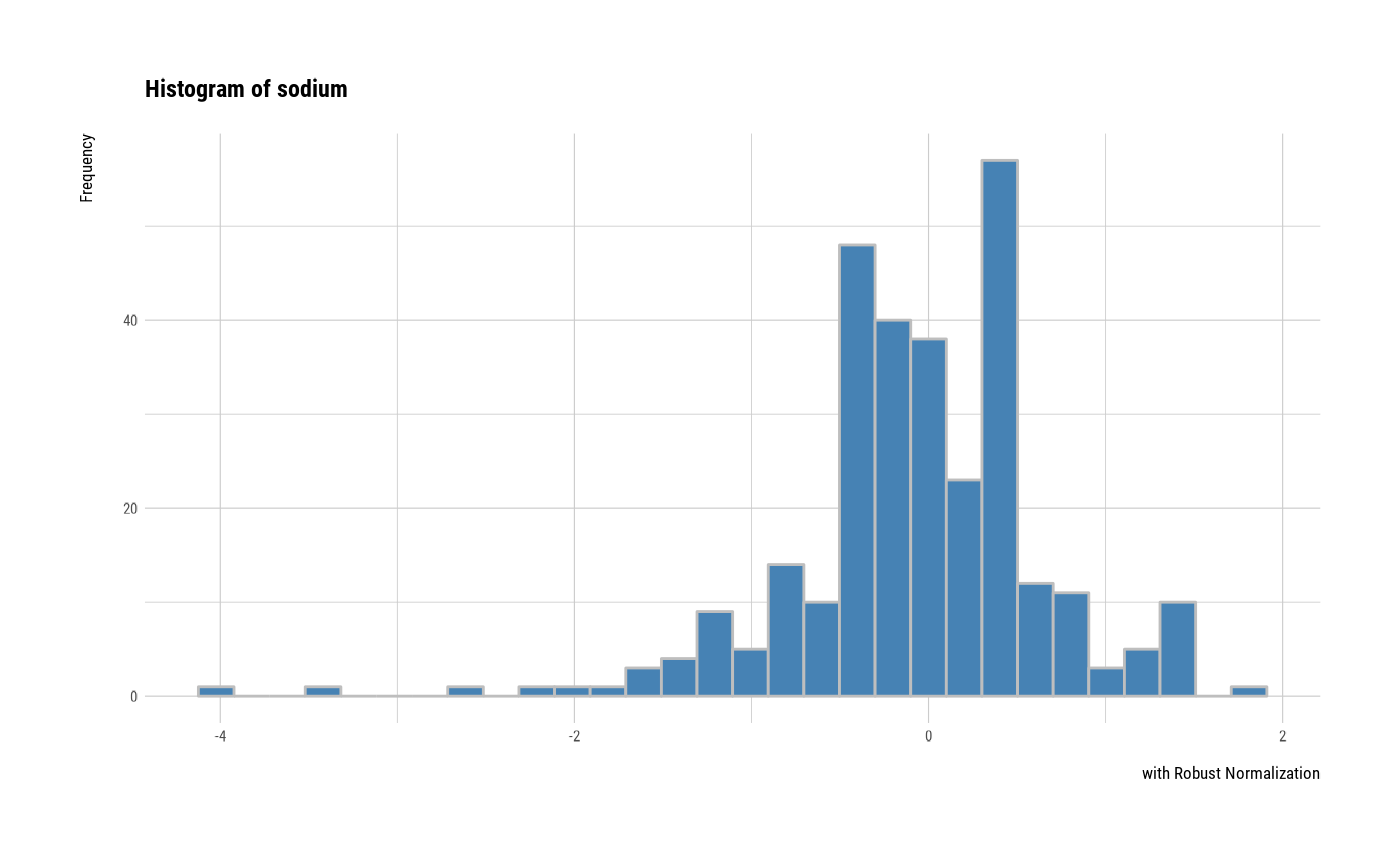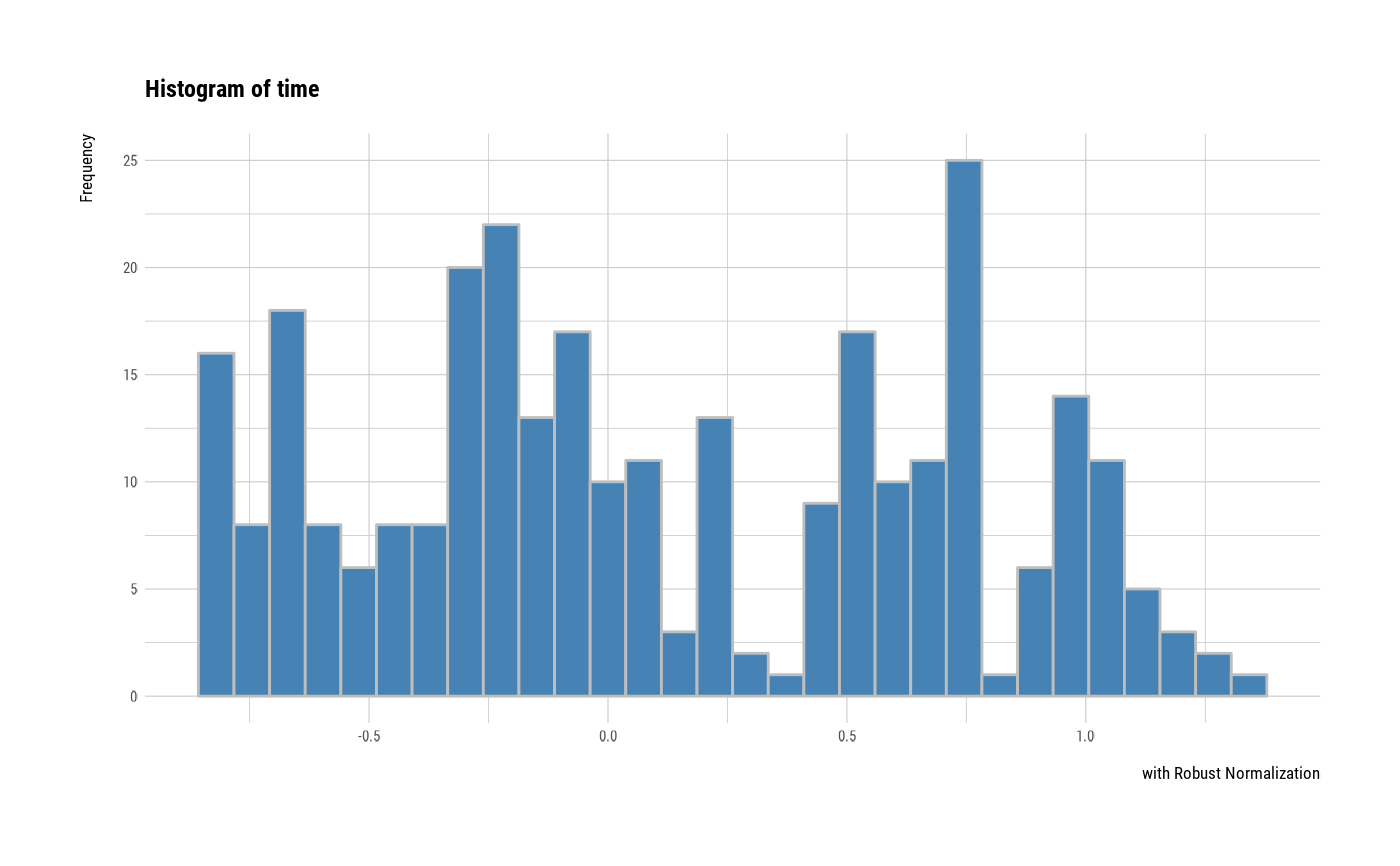# plot all variables by prompt
plot(all_var, indiv = TRUE, "hist", prompt = TRUE)# }